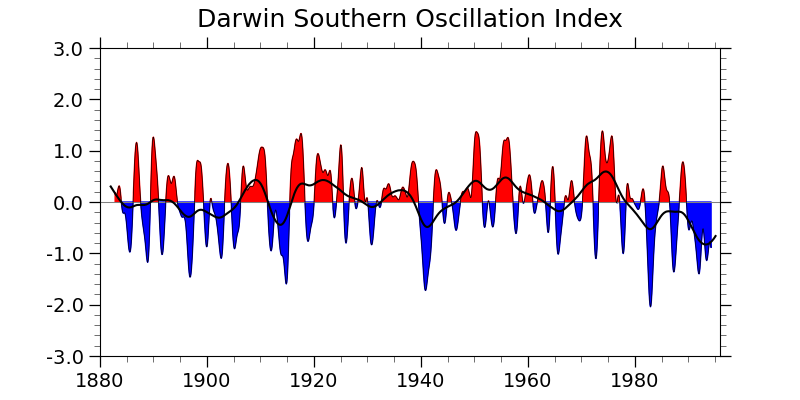# NCL_xy_5.py#

This script illustrates the following concepts:
• Draw multiple curves on an XY plot

• Drawing a Y reference line in an XY plot

• Filling the areas of an XY curve above and below a reference line

• Using named colors to indicate a fill color

• Converting dates from YYYYMM format to floats

• Creating a main title

• Setting the mininum/maximum value of the Y axis in an XY plot

See following URLs to see the reproduced NCL plot & script:

Import packages:

```import numpy as np
import xarray as xr
import matplotlib.pyplot as plt

import geocat.datafiles as gdf
import geocat.viz as gv
```

```# Open a netCDF data file using xarray default engine and load the data into xarrays
ds = xr.open_dataset(gdf.get("netcdf_files/soi.nc"))
dsoik = ds.DSOI_KET
dsoid = ds.DSOI_DEC
date = ds.date
num_months = np.shape(date)

# Dates in the file are represented by year and month (YYYYMM)
# representing them fractionally will make ploting the data easier
# This produces the same results as NCL's yyyymm_to_yyyyfrac() function
date_frac = np.empty_like(date)
for n in np.arange(0, num_months, 1):
yyyy = int(date[n] / 100)
mon = (date[n] / 100 - yyyy) * 100
date_frac[n] = yyyy + (mon - 1) / 12
```

Plot:

```# Generate figure (set its size (width, height) in inches) and axes
plt.figure(figsize=(8, 4))
ax = plt.gca()

# Plot reference line
ax.axhline(y=0, color='grey', linewidth=0.75)

# Plot data
# _labels=False prevents axis labels from being drawn
ax.plot(date_frac, dsoik, color='black', linewidth=0.5)
ax.plot(date_frac, dsoid, color='black')

# Fill above and below the 0 line
ax.fill_between(date_frac, dsoik, where=dsoik > 0, color='red')
ax.fill_between(date_frac, dsoik, where=dsoik < 0, color='blue')

# Use geocat.viz.util convenience function to add minor and major tick lines
x_minor_per_major=4,
y_minor_per_major=5,
labelsize=14)

# Use geocat.viz.util convenience function to set axes parameters
gv.set_axes_limits_and_ticks(ax,
ylim=(-3, 3),
yticks=np.linspace(-3, 3, 7),
yticklabels=np.linspace(-3, 3, 7),
xlim=(date_frac, date_frac[-1]),
xticks=np.linspace(1880, 1980, 6))

# Use geocat.viz.util convenience function to set titles and labels
gv.set_titles_and_labels(ax, maintitle="Darwin Southern Oscillation Index")

plt.show()
```Total running time of the script: ( 0 minutes 0.911 seconds)

Gallery generated by Sphinx-Gallery